ď»ż

### Introduzione

#### Abstract

En
Given a set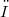$Ď‰$, a finitely additive probability measure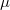$\mu$ on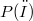$P(Ď‰)$ is considered. Let$\mu$ be "strongly" non-atomic: we prove that there exists a sequence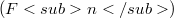$(Fn)$ of subsets of$Ď‰$ (mutually disjoint and with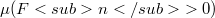$\mu ({Fn} >0)$) whose union has measure equal to an arbitrarily given đť›Ľ (with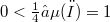$0< đť›Ľ â‰¤ \mu(Ď‰)=1$) and such that$\mu$ is countably additive on them. As a simple corollary, the following property (well-known for countably additive measures)is deduced: the range of$\mu$ is the whole interval [0,1]. In the last part of the paper, some aspects of a decomposition theorem by B. De Finetti (for an arbitrary$\mu$) are deepened.

DOI Code: §

Full Text: PDF